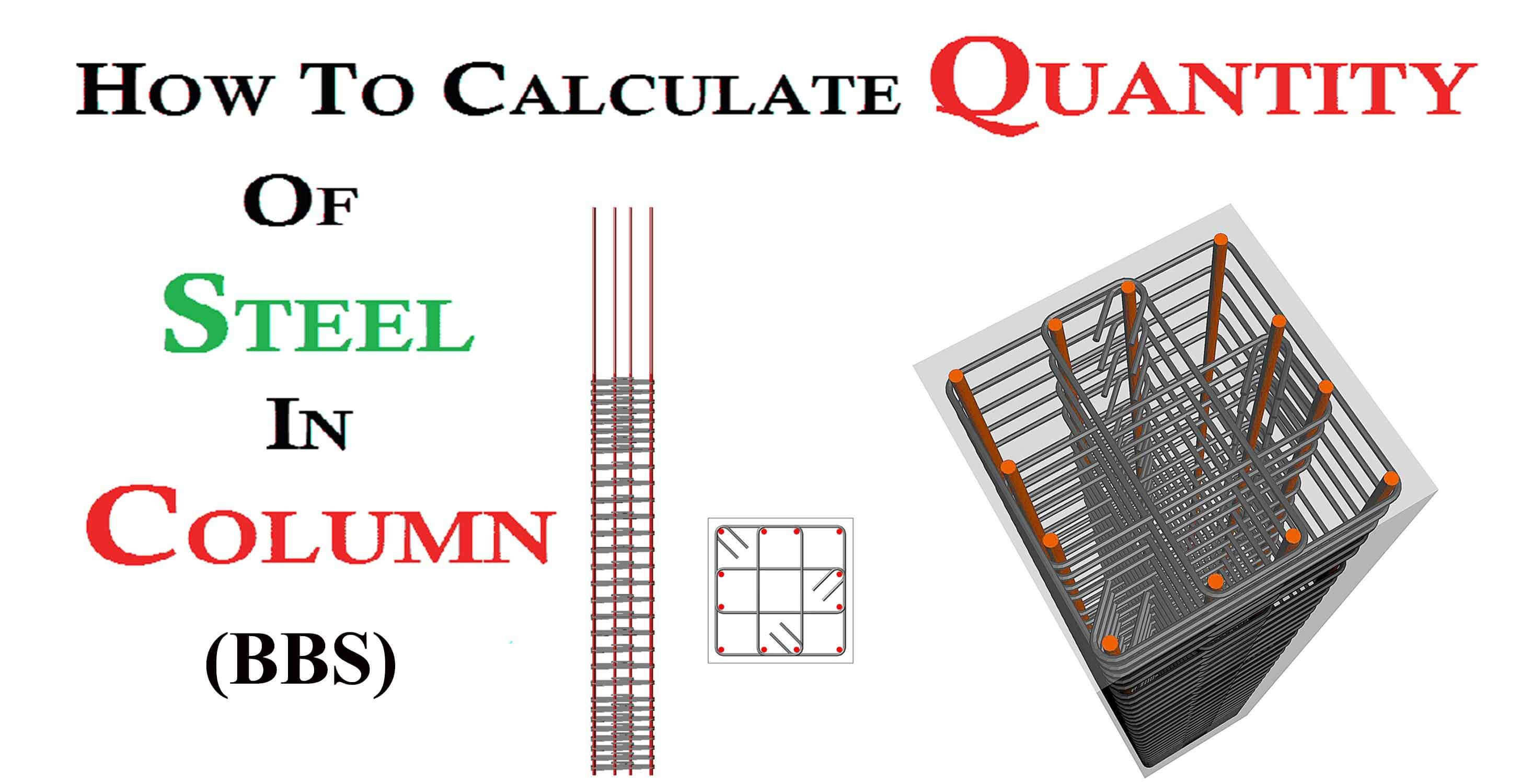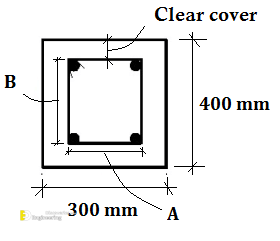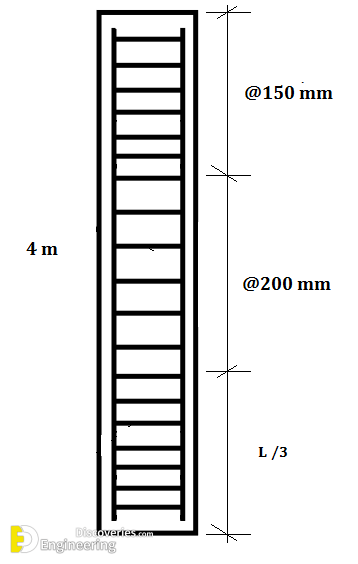# How to calculate quantity of steel to use on a column – BBS

########## Example

suppose we have a column. The height of the column is 4 m and having a cross-sectional area is 300 x 400 mm and having a 40 mm of clear cover. Six bars are going to use having a diameter of 16 mm. The diameter of the stirrup is 8 mm and having a spacing @ 150 mm and @200 mm at L/3 respectively.

###### Given Data

Height  = 4 meter

Cross section = 300 x 400 mm

Clear cover  = 40 mm.

No of vertical bars  = 6 no’s.

The diameter of the vertical bar = 16 mm.

Diameter of stirrup = 8 mm.

stirrups center to center spacing = @150 or @ 200 mm.

BBS of Column =?

###### Solution

The calculation was to proceed into two steps.

1. vertical bars calculation
2. Cutting length of stirrups

########## Step 1:Vertical Bar Calculation

Length of 1 bar = H + Ld

#Where

Ld = development lengthH = Height of column

Length of 1 bar

= 4000 mm + 40d   <where d is dia of bar>

= 4000 + 40 x 16

= 4000 + 640

4640 mm  or  4.640 m Ans..

The length one vertical bar is 4.640 m. we have total bar six bars,

Total length

READ  10 tips from a successful real estate investor in Kenya

= 6 x 4.640

27.84 m long vertical bar is required.

###### Step 2:Cutting The Length Of Stirrups

The cross-sectional area of the column is 300 mm x 400 mm

A: is the vertical cross-section area of the stirrup

B: is a horizontal cross-section area of the stirrup###### Calculation of length A

A = Horizontal Distance – 2 Side clear cover

A = 300  – 2 x clear cover

A = 300  – 2 x 40

A = 300  – 80

A = 220 mm

###### The length of B

B = Vertical distance –  2 x Top, Bottom cover

B = 400 – 2 x clear cover

B = 400 – 2 x 40

B = 400 – 80

B = 320 mm

###### No of stirrups

= 4000/3

=1333.3 mm or  1.33 mFormula = L/3 / spacing + 1

(no of stirrups in end zone)

=1333.3 / 150

= 8.8 nos say 9 nos

There are total two zones of 150 mm spacing and one zone of 200 mm spacing.

=  2 x 9

= 18 nos (at end zones)

At Mid Zone

=1333.3 / 200

= 6.6 nos say 7 nos

Total no of stirrups

= 18 + 7

= 25 nos

###### Formula

= (2 x A) + (2 x B) + hook – bend

Cutting length

= (2 x A) + (2 x B) + 2 x10d – 5 x 2d

# where

hook = 10d

bend = 5 x 2d  (we have 5 bends in one stirrup)

d =  is diameter of bar

= (2 x 220) + ( 2 x 320) + 2 x 10 x 8 – 2 x 5 x 8

= 440 + 640 + 160 – 80

1160 mm or 1.16 m

We have a total 25 nos of stirrups, which are going to use,

Total length

= 25 x 1.16

29 m long 8 mm bar.

###### Civil Notes APP

Quantity surveying,  Concrete,  Steel, Notes available in this Android App.